# Human Pose Estimation with Deep Learning

A Human Pose Skeleton represents the orientation of a person in a graphical format. Essentially, it is a set of coordinates that can be connected to describe the pose of the person. Each co-ordinate in the skeleton is known as a part (or a joint, or a keypoint). A valid connection between two parts is known as a pair (or a limb).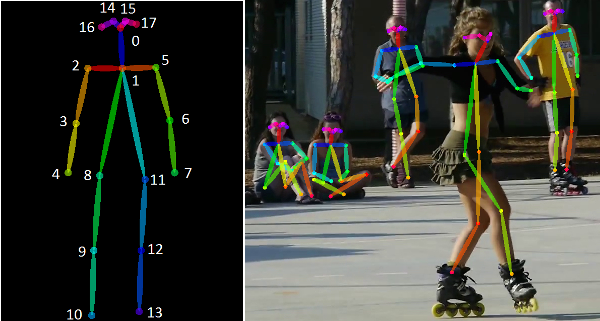For a more detailed list of huamn pose estimation methods, please refer to awesome-human-pose-estimation

# Top-down vs Button-up

There are generally two categories of approaches:

• top-down: incorporate a person detector first, followed by estimating the parts and then calculating the pose for each person.
• bottom-up: detect all parts in the image (i.e. parts of every person), followed by associating/grouping parts belonging to distinct persons.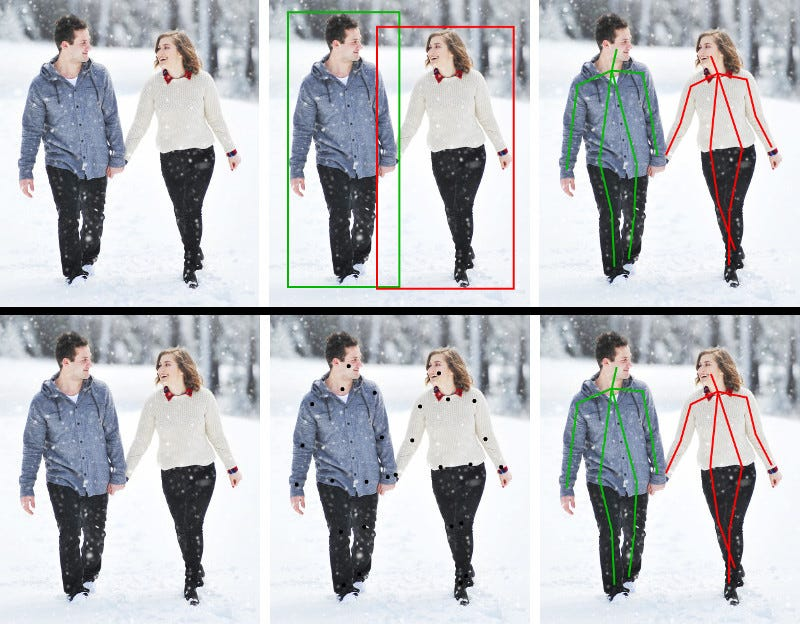# Method

## OpenPoseDeepPose was the first major paper that applied Deep Learning to Human pose estimation. It achieved SOTA performance and beat existing models. In this approach, pose estimation is formulated as a CNN-based regression problem towards body joints. They also use a cascade of such regressors to refine the pose estimates and get better estimates. One important thing this approach does is the reason about pose in a holistic fashion, i.e even if certain joints are hidden, they can be estimated if the pose is reasoned about holistically.

The model consisted of an AlexNet backend (7 layers) with an extra final layer that outputs 2k joint coordinates and The model is trained using a $\ell_2$ loss for regression.

An interesting idea this model implements is refinement of the predictions using cascaded regressors. Initial coarse pose is refined and a better estimate is achieved. Images are cropped around the predicted joint and fed to the next stage, in this way the subsequent pose regressors see higher resolution images and thus learn features for finer scales which ultimately leads to higher precision.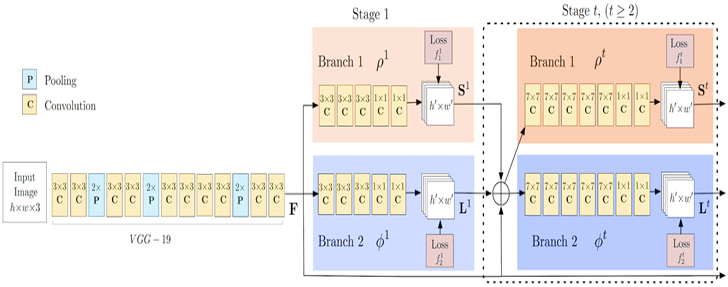## Efficient Object Localization Using Convolutional Networks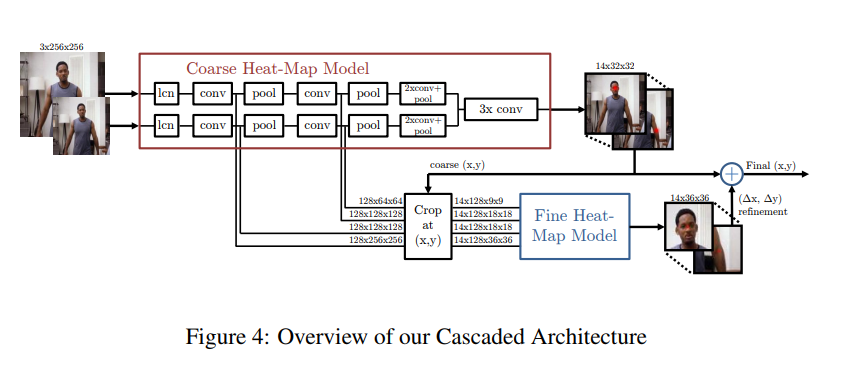This approach generates heatmaps by running an image through multiple resolution banks in parallel to simultaneously capture features at a variety of scales. The output is a discrete heatmap instead of continuous regression. A heatmap predicts the probability of the joint occurring at each pixel. This output model is very successful and a lot of the papers that followed predict heatmaps instead of direct regression.

A multi-resolution CNN architecture (coarse heatmap model) is used to implement a sliding window detector to produce a coarse heatmap output.

The main motivation of this paper is to recover the spatial accuracy lost due to pooling in the initial model. They do this by using an additional ‘pose refinement’ ConvNet that refines the localization result of the coarse heat-map. However, unlike a standard cascade of models, they reuse existing convolution features. This not only reduces the number of trainable parameters in the cascade but also acts as a regularizer for the coarse heat-map model since the coarse and fine models are trained jointly.

## Convolutional Pose Machines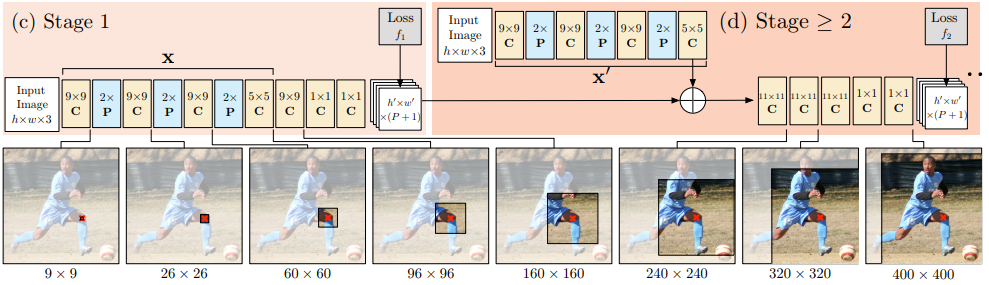A pose machine consists of an image feature computation module followed by a prediction module. Convolutional Pose Machines are completely differentiable and their multi-stage architecture can be trained end to end. One of the main motivations of this paper is to learn long range spatial relationships and they show that this can be achieved by using larger receptive fields.

A CPM can consist of > 2 Stages, and the number of stages is a hyperparameter. (Usually = 3). Stage 1 is fixed and stages > 2 are just repetitions of Stage 2. Stage 2 take heatmaps and image evidence as input. The input heatmaps add spatial context for the next stage.

## Human Pose Estimation with Iterative Error Feedback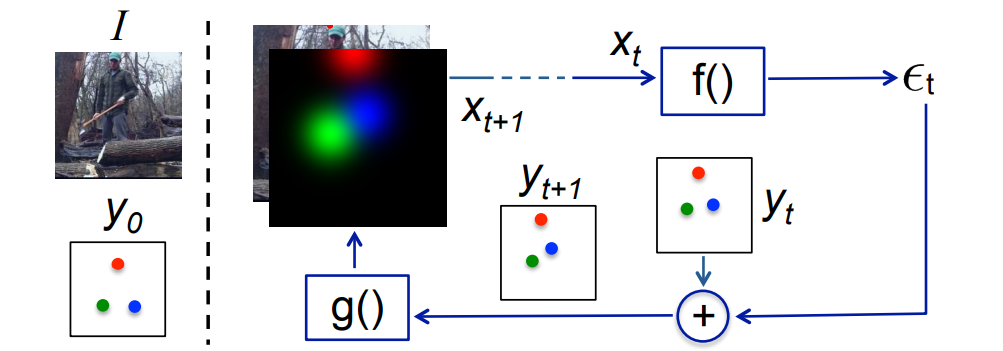Instead of directly predicting the outputs in one go, they use a self-correcting model that progressively changes an initial solution by feeding back error predictions, and this process is called Iterative Error Feedback (IEF).

## Stacked Hourglass Networks for Human Pose Estimation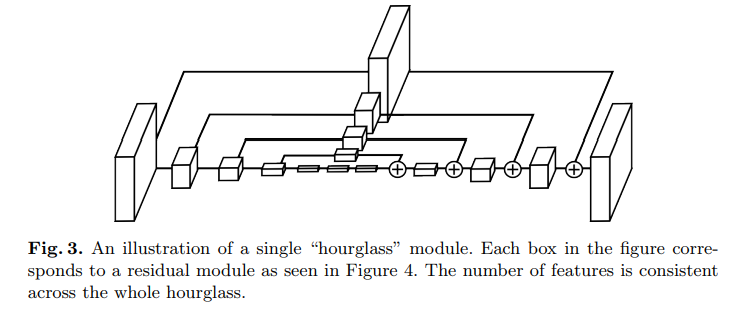This is a landmark paper that introduced a novel and intuitive architecture and beat all previous methods. It’s called a stacked hourglass network since the network consists of steps of pooling and upsampling layers which looks like an hourglass, and these are stacked together. The design of the hourglass is motivated by the need to capture information at every scale. While local evidence is essential for identifying features like faces hands, a final pose estimate requires global context.

## Simple Baselines for Human Pose Estimation and TrackingThe network structure is quite simple and consists of a ResNet + few deconvolutional layers at the end. Mean Squared Error (MSE) is used as the loss between the predicted heatmaps and targeted heatmaps.

The OpenPose network first extracts features from an image using the first few layers (VGG-19 in the above flowchart). The features are then fed into two parallel branches of convolutional layers. The first branch predicts a set of 18 confidence maps, with each map representing a particular part of the human pose skeleton. The second branch predicts a set of 38 Part Affinity Fields (PAFs) which represents the degree of association between parts.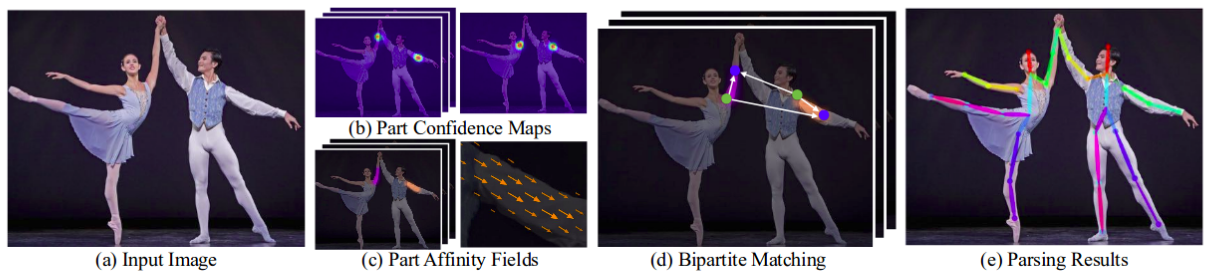Successive stages are used to refine the predictions made by each branch. Using the part confidence maps, bipartite graphs are formed between pairs of parts. Using the PAF values, weaker links in the bipartite graphs are pruned. Through the above steps, human pose skeletons can be estimated and assigned to every person in the image.

## DeepCut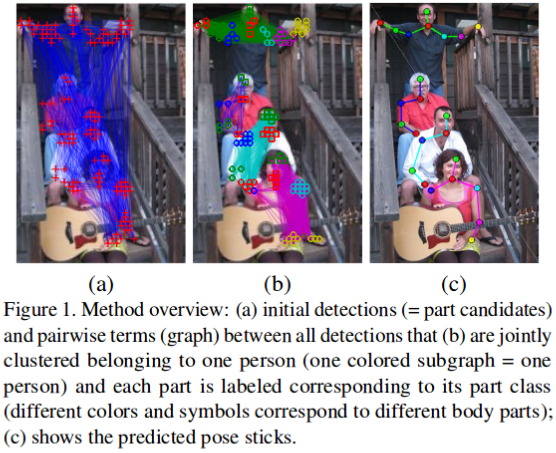DeepCut is a bottom-up approach for multi-person human pose estimation. The authors approached the task by defining the following problems:

• Produce a set of D body part candidates (through a Faster RCNN or a Dense CNN). This set represents all possible locations of body parts for every person in the image. Select a subset of body parts from the above set of body part candidates.
• Label each selected body part with one of C body part classes. The body part classes represent the types of parts, such as “arm”, “leg”, “torso” etc.
• Partition body parts that belong to the same person.

The above problems were jointly solved by modeling it into an Integer Linear Programming (ILP) problem.

## AlphaPose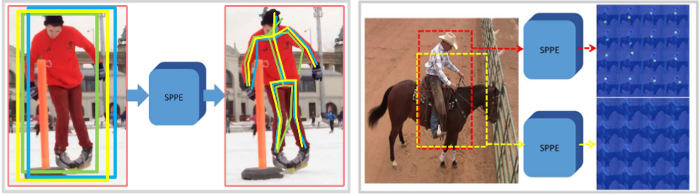AlphaPose is a popular top-down method of Pose Estimation. The authors posit that top-down methods are usually dependent on the accuracy of the person detector, as pose estimation is performed on the region where the person is located. Hence, errors in localization and duplicate bounding box predictions can cause the pose extraction algorithm to perform sub-optimally.

To resolve this issue, the authors proposed the usage of Symmetric Spatial Transformer Network (SSTN) to extract a high-quality single person region from an inaccurate bounding box. A Single Person Pose Estimator (SPPE) is used in this extracted region to estimate the human pose skeleton for that person.

A Spatial De-Transformer Network (SDTN) is used to remap the estimated human pose back to the original image coordinate system. Finally, a parametric pose Non-Maximum Suppression (NMS) technique is used to handle the issue of redundant pose deductions.

Furthermore, the authors introduce a Pose Guided Proposals Generator to augment training samples that can better help train the SPPE and SSTN networks. The salient feature of RMPE is that this technique can be extended to any combination of a person detection algorithm and an SPPE.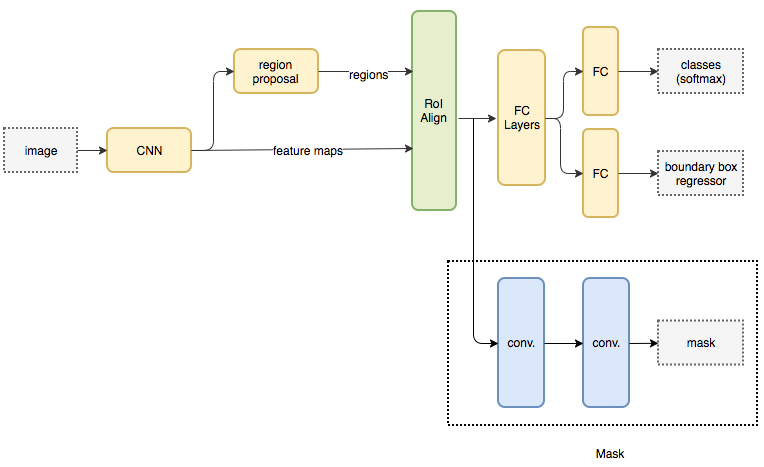Mask RCNN is a popular architecture for performing semantic and instance segmentation. The model parallelly predicts both the bounding box locations of the various objects in the image and a mask that semantically segments the object. The basic architecture can be quite easily extended for human pose estimation.

## HRNetMost of the previous papers went from a high → low → high-resolution representation. HRNet maintains a high-resolution representation throughout the whole process and this works very well. The architecture starts from a high-resolution subnetwork as the first stage, and gradually adds high-to-low resolution subnetworks one by one to form more stages and connect the multi-resolution subnetworks in parallel. Repeated multi-scale fusions are conducted by exchanging information across parallel multi-resolution subnetworks over and over through the whole process.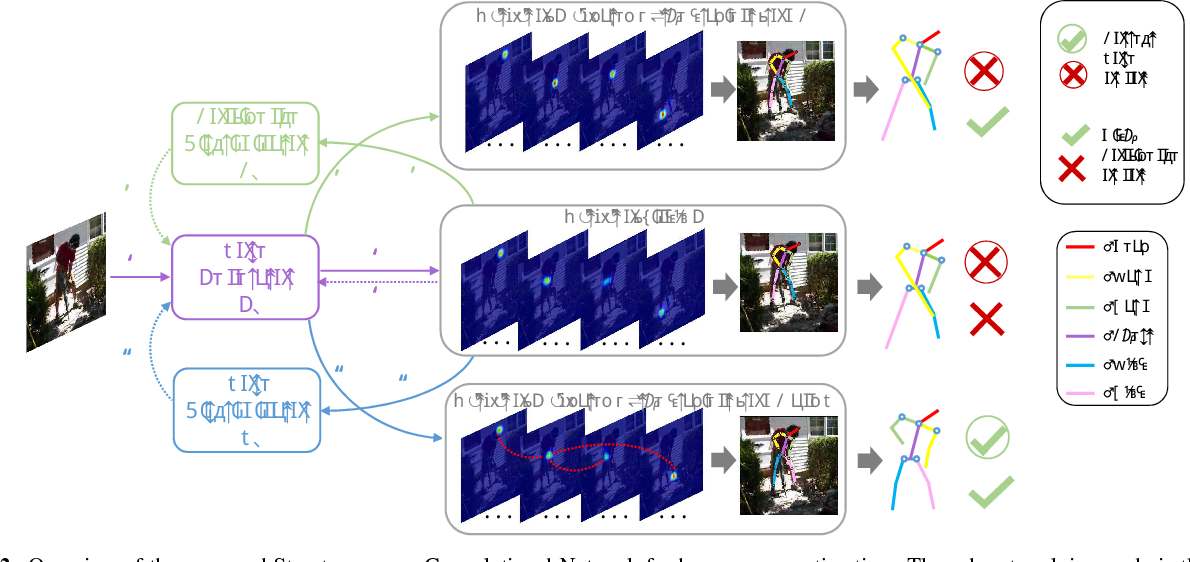Adversarial PoseNet considers joint occlusions and overlapping upon human bodies for human pose estimation in monocular images. Under these circumstances, biologically implausible pose predictions may be produced. A novel structure-aware convolutional network to implicitly take such priors into account during training of the deep network, under GAN framework. The generator G is designed in a stacked multi-task manner to predict poses as well as occlusion heatmaps. Then, the pose and occlusion heatmaps are sent to the discriminators to predict the likelihood of the pose being real.

Two disrcrimiantors are introduced one for whether the generated pose is “real” (reasonable as a body shape), which is based on encoder-decoder architecture with skip connections and the other for whether the generator has strong confidence in locating the body parts,

# Common Evaluation Metrics

• Percentage of Correct Parts - PCP: A limb is considered detected (a correct part) if the distance between the two predicted joint locations and the true limb joint locations is less than half of the limb length (Commonly denoted as PCP@0.5).
• Percentage of Correct Key-points - PCK: A detected joint is considered correct if the distance between the predicted and the true joint is within a certain threshold. The threshold can either be:
• PCKh@0.5 is when the threshold = 50% of the head bone link
• PCK@0.2 == Distance between predicted and true joint < 0.2 * torso diameter
• Sometimes 150 mm is taken as the threshold.
• Alleviates the shorter limb problem since shorter limbs have smaller torsos and head bone links.
• PCK is used for 2D and 3D (PCK3D). Again, the higher the better.
• Percentage of Detected Joints - PDJ: A detected joint is considered correct if the distance between the predicted and the true joint is within a certain fraction of the torso diameter. PDJ@0.2 = distance between predicted and true joint < 0.2 * torso diameter.
• Object Keypoint Similarity (OKS) based mAP: similar as Intersecion over Union (IoU). $$\frac{\sum_i{e^{-\frac{d_i^2}{2s^2k_i^2}}\delta(v_i\gt0)}}{\sum_i{\delta(v_i\gt0)}}$$, where Where $d_i$ is the Euclidean distance between the detected keypoint and the corresponding ground truth, $v_i$ is the visibility flag of the ground truth, s is the object scale, and $k_i$ a per-keypoint constant that controls falloff.
Written on May 5, 2019